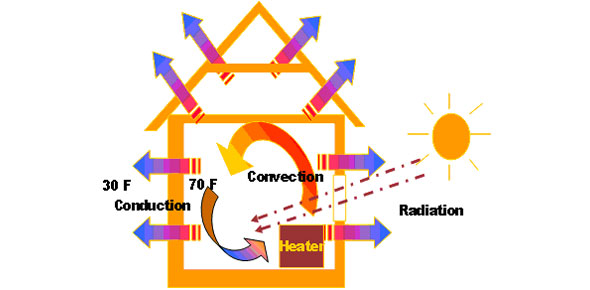# 2.06 Heat, Heat Transfer And Energy Conversions

10 Questions | Total Attempts: 158SettingsThis is a quiz on heat, heat transfer and energy conversions

• 1.
How does thermal energy naturally travel?
• A.

From a source that has less energy to a source with more energy

• B.

From a source that has more energy to a source of less energy

• C.

It doesn't travel anywhere

• D.

It just keeps traveling

• 2.
Heat will do what if not contained?
• A.

• B.

Stay in one area

• C.

Just disappear

• D.

Go cold

• 3.
The transfer of heat between substances that are in direct contact with each other is called what?
• A.

• B.

Convection

• C.

Conduction

• D.

Heat Transfer

• 4.
• A.

Only in a vacuum

• B.

Only in a medium

• C.

Can travel and interact with a medium or in a vacuum

• D.

Does not travel

• 5.
What is Convection?
• A.

The transfer of heat between substances that are in direct contact with each other

• B.

An electromagnetic wave that travels through space and gets warmed

• C.

Is the distribution of heat by way of moving particles.

• D.

Is another name for a microwave

• 6.
Energy cannot be what?
• A.

Created

• B.

Destroyed

• C.

Created or destroyed

• D.

Lost

• 7.
What are the two forms of Mechanical energy?
• A.

Potential and Kinetic energy

• B.

Chemical and Potential energy

• C.

Electrical and Chemical energy

• D.

Sound and Thermal energy

• 8.
Where is Nuclear energy found?
• A.

In the nuclei of atoms

• B.

In electrical waves

• C.

Stored in the bonds of different compounds

• D.

In electromagnetic waves

• 9.
What energy cannot be transmitted in a vacuum?
• A.

Light

• B.

Electrical

• C.

Thermal

• D.

Sound

• 10.
What can be transferred from one object to another or converted from one form to another?
• A.

Sounds

• B.

Electricity

• C.

Energy

• D.

Light

Related TopicsBack to top